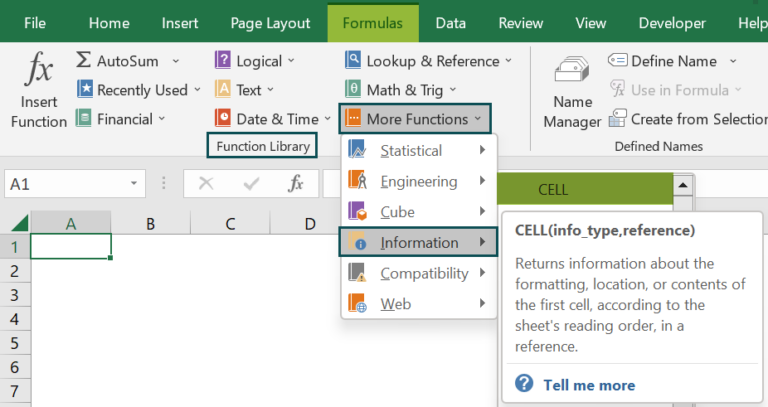# CELL Function In ExcelArticle byExcelMojo Team## What Is CELL Function In Excel?

The CELL function in Excel extracts and returns the requested information about a cell value regarding its contents, formatting, location, etc. In a large dataset, the function helps identify numeric values before proceeding with any calculations. The Excel CELL function is an inbuilt Information function, so we can insert the formula from the “Function Library” or enter it directly in the worksheet. For example, we will use the CELL function to find the cell address for the value in column A.

Select cell B2, enter the formula =CELL(“address”,A2), and press “Enter”. The result is ‘\$A\$2’, as shown below, indicating that the selected cell’s cell address is A2.

###### Key Takeaways
• The CELL function in Excel helps users find the needed facts or information w.r.t a cell value in a given dataset.
• The info_type tells what kind of information is required, such as its cell address, formatting [color, format, etc.], location [column, row, etc.], etc.
• We can see the “Info-type” table and the “Format Code” table provided, at the start of the article, to comprehend what results or output to expect when the function is executed.
• For the reference argument, we must select the cells in a dataset or a cell range, apply the CELL formula, and extract the required details.

### CELL() Excel Formula

The syntax of the CELL Excel Formula is,

The arguments of the CELL Excel Formula are,

• info_type: It is a mandatory argument. It is the cell information that is to be returned.

Info Type value – The table below contains the Info type and its description.

Cell Format Codes

• reference: It is an optional argument. It is the cell from which the information is collected.

### How To Use CELL Function In Excel?

We can use the CELL function in Excel in 2 ways, namely,

1. Access from the Excel ribbon.
2. Enter in the worksheet manually.

#### Method #1 – Access from the Excel ribbon

Choose an empty cell for the output → select the “Formulas” tab → go to the “Function Library” group → click the “More Functions” option drop-down → click the “Information” option right arrow → select the “CELL” function, as shown below.

The “Function Arguments” window appears. Enter the arguments in the “Info_type” and “Reference” fields → click “OK”, as shown below.

#### Method #2 – Enter in the worksheet manually

1. Select an empty cell for the output.
2. Type =CELL( in the selected cell. [Alternatively, type =C and double-click the CELL function from the list of suggestions shown by Excel.]
3. Enter the arguments as cell values or cell references and close the brackets.
4. Press the “Enter” key.

Let us take a basic example to understand this function.

We will find the formatting of the cell values using CELL function in Excel.

In the table, the data is,

• Column A contains the Data.
• Column B contains the Result.

The steps to find the required details using the CELL formula are,

1. Select cell B2, and enter the formula =CELL(“format”, i.e., the format value of the cell.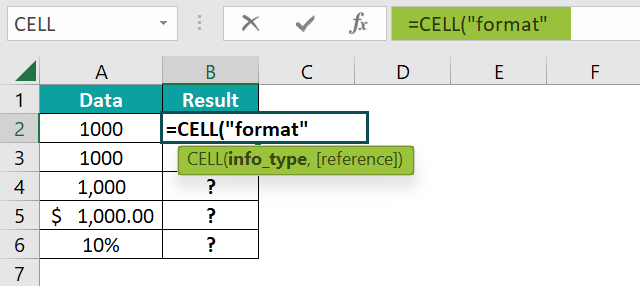2. Enter the value of ‘reference’ as A2, and close the brackets. The complete formula is =CELL(“format”,A2).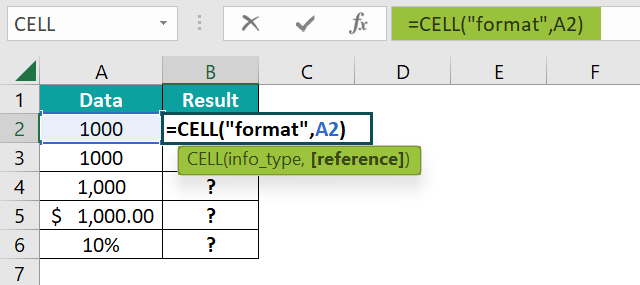3. Press the “Enter” key. The results in cell B2, as shown below.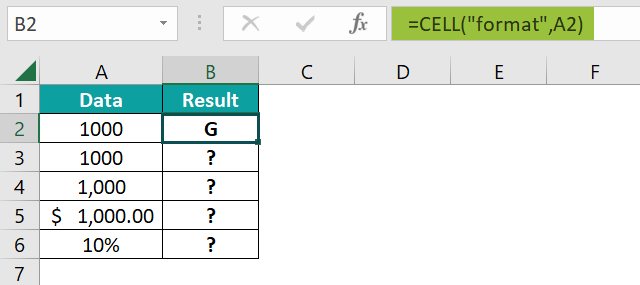4. Drag the formula from cell B2 to B6 using the fill handle. The output is shown below.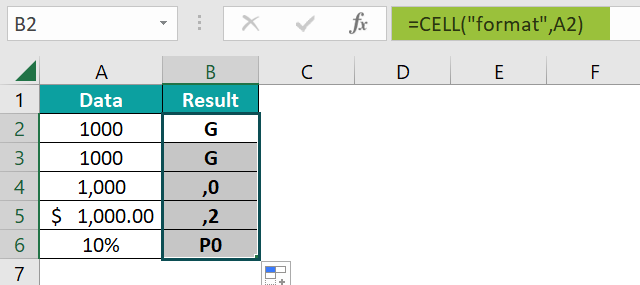### Examples

We will understand some advanced scenarios using the CELL function in Excel examples.

#### Example #1

We will find the column cell reference of the cell values using the CELL function in Excel.

In the table, the data is,

• Column A contains the Data.
• Column B contains the Result.

The steps to find the required details using the CELL formula are,

• Step 1: Select cell B2, enter the formula =CELL(“col”,A2), and press “Enter”. The result is ‘1’, as shown below, indicating the column number of the selected cell.
• Step 2: Drag the formula from cell B2 to B5 using the fill handle. The output is shown below.

#### Example #2

We will find the prefix of the cell values using the CELL function in Excel.

In the table, the data is,

• Column A contains the Data.
• Column B contains the Result.

The steps to find the required details using the CELL formula are,

• Step 1: Select cell B2, enter the formula =CELL(“prefix”,A2), and press “Enter”. The result is ’.
• Step 2: Drag the formula from cell B2 to B5 using the fill handle. The output is shown below.

#### Example #3

We will find the color of the cell values using the CELL function in Excel.

In the table, the data is,

• Column A contains the Data.
• Column B contains the Result.

The procedure to find the required details using the CELL formula is,

Enter the formula =CELL(color, A2) in cell A2, =CELL(“abc”,A3) in cell A3, and =CELL(6,A4) in cell A4. Press the “Enter” key every time the formula is entered.

The result in cell B2 is a “#NAME?” error”, and in cells B3 and B4 are “VALUE!” errors, as shown in the image above. Column C is for our reference.

### Important Things To Note

• The “#NAME?” error occurs when the “info_type” arguments are text values that are not enclosed in quotes (“ ”) and are not valid cell references.
• The “#VALUE!” error occurs when,
• The “info_type” value is less than one or is greater than the given number of values.
• The “info_type” argument value is non-numeric.

1. What does the CELL function in Excel mean?

The CELL function returns the information about cell references by taking 2 arguments, such as what info is needed, whether the cell values location, format, address, and which cell value to find the required details.

The Formula of the CELL function is =CELL(info_type,reference)

2. How to insert the CELL function in Excel?

We can insert Excel CELL Function as follows:
1. Select an empty cell for the output.
2. Type =CELL( in the selected cell. [Alternatively, type =C and double-click the CELL function from the list of suggestions shown by Excel.]
3. Enter the arguments as cell values or cell references and close the brackets.
4. Press the “Enter” key.

For example, we will find the cell content using the CELL function for the following values.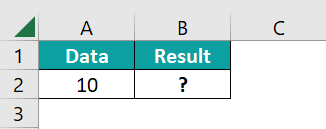Select cell B2, enter the formula =CELL(“contents”,A2), and press the “Enter” key.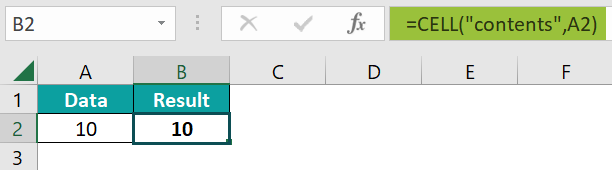The result is ‘10’, as shown above.

3. Where is the CELL function in Excel?

The CELL Excel Function is found in the “Formulas” tab, as shown below.

Choose an empty cell for the output → select the “Formulas” tab → go to the “Function Library” group → click the “More Functions” option drop-down → click the “Information” option right arrow → select the “CELL” function, as shown below.